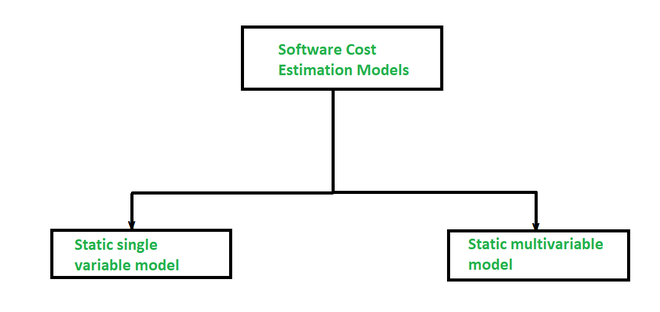# Software Cost Estimation Models in Software Planning

• Last Updated : 26 Apr, 2021

In starting a new software project, it is important to know how much will it cost in developing and how much development time will it take to complete. These evaluations are needed before development is started and conveyed to the team. The software industry has inconsistently defined and explained metrics or atomic units of measure, data from real and actual projects are largely and highly suspect in terms of consistency and comparability. There are many questions as debates among developers and vendors of software cost estimation models and tools. The various estimation procedures that have been developed and have the following common characteristics are given below:

• The scope of the software project must be created before start developing the software.
• Metrics like FP or LOC are used for assessing the software.
• To achieve the targeted cost & schedule estimate, several things arise.
• Obtain one or more cost and effort of a project.

Below are the two models in estimating the cost of a software project:In a static model, a single variable is grabbed as a key element for calculating the cost and effort whereas, In a dynamic model, all variables are connected with each other, and there is no primary variable.

1. Static Single Variable model- The Methods using this model utilizes an equation to get the desired values such as cost, time, and effort, etc. And these all depend on the same variable used as a predictor like, size. Below is the example of the most common equation:

C = aLb

Where C is cost, L is size and a, b are constants.

We have an example of the static single variable model, i.e SEL model which is used for estimating software production. The equation of this model is given below:

E = 1.4L0.93

DOC = 30.4L0.90

D = 4.6L0.26

Where E is in Person-months, DOC i.e, documentation is in the number of pages, D is duration which is months.

2. Static Multivariable model- These models are also known as multivariable models. This model is often based on the first equation and actually depends on several variables representing different aspects of the software development environment.

Equations are:

E = 5.2L0.91

D = 4.1L0.36

Where E is in Person-months, D is duration which is months.

My Personal Notes arrow_drop_up